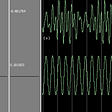# Number partitioning using coin change

`Input: 6 and K= 2Output: 24Explanation: There are 4 valid partitions.{6}{4 , 2}{3, 3}{2, 2, 2}Therefore the total sumwould be 6+4+2+3+3+2+2+2=24`
`import java.io.*;import java.util.*;class Main {  //function to find number of valid  //partitons of n    static int partition(int n, int k){      //create a dp table on n+1 elements      int[] dp = new int[n+1];            //initilaise 0th index with 1       // as there is only 1 way to partition 0      dp=1;            //first loop indicates the numbers available to      // us for partitioning j integer      // Example for i= 4 , (k= 4) we have      // 2, 3, 4 numbers to partiton j Integer      for(int i=k ; i<n+1 ; i++){        for(int j=1 ; j<n+1 ; j++){        // Only when integer to be partitioned is         //greater than the available numbers        if(i<=j){          //First exclude the number i to partition j          // Second include the number i to partition j          // add them both           dp[j]= dp[j]+dp[j-i];                   }        }      }       // The laSt element of array is the answer      return dp[n];    }     // driver function    public static void main (String[] args) {      int n= 6;      int k=2;      // Calling partition to calculate      // number of valid partitions      int ans= partition(n , k);      // sum is number of valid partitions * n      System.out.println("The aggregate sum is "+ans*n);    }}`

--

--

--

## More from Abhinay Gupta

Love podcasts or audiobooks? Learn on the go with our new app.

## Android’s Database## 1-click integration of Matic Widget ERC20 contracts## Let’s fry up something nice.## Diving into Java (with strong JavaScript and Python background) Part 1 (Naming Conventions and…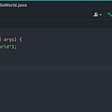## Leetcode-stack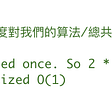## WOT: simple distributed leader consensus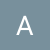## Add Math Equations to PowerPoint in Java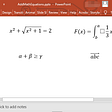## Object-Oriented Programming Explained## Why Learning Programming Takes Time## Generic FIR Filter Using Floating-Point IP in Vivado